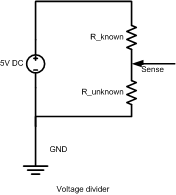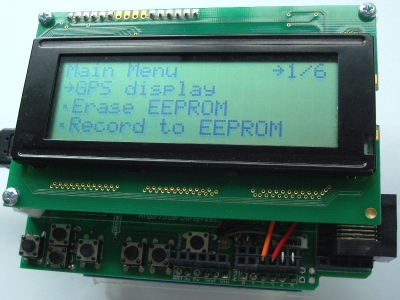# Using commercial temperature probes for digital thermometer

I've gotten some temperature probes from an oneida 31161 digital thermometer. I want to them it to build a digital thermometer, preferably being able to measure from 3 probes at once. I am wondering if it is feasible to do it this way, or if I am better off using a DS18B20 and following one of the many project guides I have seen? I do plan to use this for liquid temp measurements, so the sealed and compact commercial probes are attractive.

Thanks

If you have a link to a datasheet it can be figured out... The advantage of commercial probes is that they are (often) calibrated.

Thermistors have a funny temperature vs. resistance curve. If you calibrate them you can get fairly accurate temperatures with just a resistor and analog input. It's a pain in the ass.You can get fairly close by making note of the analog readings at a few temperatures across the range you want to measure (verified with a known good thermometer) and interpolate between points. If you are very lucky your three probes can all use the same table.

Measure the probe resistance at room temperature. Use a pull-up (or pull down) resistor of about 1/2 that value. Use the probe to pull down (or pul up) the analog input line.

You can get fairly close by making note of the analog readings at a few temperatures across the range you want to measure (verified with a known good thermometer) and interpolate between points.

See playground - Arduino Playground - MultiMap -

I measured the resistance at a few different temperatures and it looks like it is pretty linear when graphed on a log scale. Could this be as easy as programing the arduino to do the math and output the result?

If you can find a function that works for your thermistor over the range of temperatures you are trying to measure then that should be fine. I know that some spreadsheet programs can fit a polynomial to a set of points. That might produce a usable function.

This will be my first homegrown project with the arduino. So far I've just plugged it into things that already had plans and code.
As far as I understand, the arduino can detect voltage changes, but not resistance changes. Can I not just apply voltage to one pin of the probe and measure the voltage with the arduino at the other?

Can you please explain how I would arrange the pull-up resistor that you were talking about?

And in case it matters, the resistance range of the probe is from about 13.5kohm (at boiling) to about 700kohm at freezing. The relationship is linear when I take the log of the resistance.

That's about right. Typically a thermistor is called a 100Kohm thermistor if its resistance is 100Kohm at 25DegC. You should use a log function and a few constants (easy if you have a calibrated thermometer on the side). Here is my tutorial:

Use the simplified version of formulaIt also shows you why you get a linear relation if you take the log of resistance

You just need two temperature points, one preferentially at 25DegC instead of 0DegC but you did the measurement already so use it and another one as far as possible from it, maybe at water boiling point. Then you can solve R1 and B and use the formula for any temperature.

If you used the right temperature scale and don't miss a negative sign like I did in my first two tries, you will get B=4022 and R1=203.6Kohm, so it's a 200Kohm thermistor with around B=4020(Kelvin)

This will be my first homegrown project with the arduino. So far I've just plugged it into things that already had plans and code.
As far as I understand, the arduino can detect voltage changes, but not resistance changes. Can I not just apply voltage to one pin of the probe and measure the voltage with the arduino at the other?

Can you please explain how I would arrange the pull-up resistor that you were talking about?

And in case it matters, the resistance range of the probe is from about 13.5kohm (at boiling) to about 700kohm at freezing. The relationship is linear when I take the log of the resistance.

I seem to be in the ball park of your estimates. B=4140 and at 297K R1=230Kohm

Would I be able to use your sample code, substituting my values, and get this to work?

Further, does the arduino have only one analog input? What would be the best way to use 3-4 probes? Should I put them on a manual switch, or is there a way that I could cycle through them? It would be nice to eventually have a 4 digit display that would display "A100", "B 25", etc. Ideally, I would be able to have a manual switch that I could select one of the probes, and then another setting that would cycle through the probes.

I seem to be in the ball park of your estimates. B=4140 and at 297K R1=230Kohm

Would I be able to use your sample code, substituting my values, and get this to work?

Further, does the arduino have only one analog input? What would be the best way to use 3-4 probes? Should I put them on a manual switch, or is there a way that I could cycle through them? It would be nice to eventually have a 4 digit display that would display "A100", "B 25", etc. Ideally, I would be able to have a manual switch that I could select one of the probes, and then another setting that would cycle through the probes.

Sure, you can use the formula on my blog for your estimate. I strongly suggest you find the spec sheet of the thermistors for better reference or, measure resistance at 25DegC for better estimate.

Arduino has 6 analog inputs, A0-A5 so you don't have to use any switches. For display, I suggest a character LCD. It's very easy to use. Take a look at my library and hardware to see if you can make use of them to generate an interactive interface on arduino:

I made about 6 measurements per unit and plotted them in excel. It calculated a linear best fit with an R^2 of .995, over the entire range that I will be using this.

Someone previously mentioned using a pull-down resistor of 1/2 the value of the thermister resistance at room temp. What is the reasoning for that value, and what exactly is the function of that resistor?

Also, I am looking to leave this project as a unit, so I don't really want to sink a lot of money into shields, nor do I plan to leave my uno in there. Is it difficult to wire up a 20x4 LCD, do I need anything else (controller chip)?

If you just connect a variable resistor from +5 to an analog input pin it will always read 1023 no matter how you set it.

If you just connect a variable resistor from Ground to an analog input pin it will always read 0 no matter how you set it.

If you connect a variable resistor from +5 to the an analog input pin AND connect a fixed resistor from the same analog input pin to Ground the reading you get will depend on the value of the variable resistor. As the resistance goes down the voltage will get closer to +5 (1023). As the resistance goes up the voltage will get closer to Ground (0). It will never get to 1023 or 0 so the value of the fixed resistor determines how close it gets to each. I thought a value about 1/2 the room temperature value of the thermistor would be about right but any value will get you a range of readings.

Thanks for the info. Out of curiosity, and forgive me if this is obvious, how does the reading of 1023 relate to 5V? Is that just a maximum reading?

The A/D converter measures the input against a reference voltage (5V by default) and produces a 10-bit unsigned number (0 to 1023) to express the relative voltage. An input voltage of half the reference will give you a value of 512.

I made about 6 measurements per unit and plotted them in excel. It calculated a linear best fit with an R^2 of .995, over the entire range that I will be using this.

Someone previously mentioned using a pull-down resistor of 1/2 the value of the thermister resistance at room temp. What is the reasoning for that value, and what exactly is the function of that resistor?

Also, I am looking to leave this project as a unit, so I don't really want to sink a lot of money into shields, nor do I plan to leave my uno in there. Is it difficult to wire up a 20x4 LCD, do I need anything else (controller chip)?

Please refer to my tutorial again for circuit diagram. You need to build something called voltage divider to chain a thermistor and a fixed resistor in series and sense the mid-point between the two to calculate for resistance. I have sample code on the tutorial too (out of one student group that did this in my class, and I straightened out any kinks already).You need to choose the right fixed resistor for optimal accuracy. First determine your range of temperature you measure first, then find the middle of that range, and pick a fixed resistor that has the same resistance as the thermistor if it were at the middle of the temperature range. IE. if your temperature measurement is around 25DegC and you are using a 10Kohm thermistor, you need a 10Kohm fixed resistor in series to the thermistor. If you use a say 200Kohm thermistor with R1=200Kohm and B=4022K (use formula 2 and 3), and your temperature range is -30DegC to 50DegC, so center is 10DegC, then you need a fixed resistor that matches the thermistor resistance at 10DegC, which is 409Kohm according to formula 2. So you either use 390Kohm or 430Kohm as both are standard values easy to obtain.

About display, it's easy. I do warn you the following is designed by me and being sold at a store